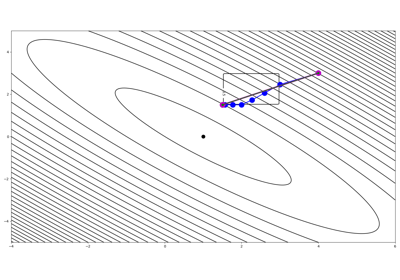# pyproximal.ProxOperator#

Common interface for proximal operators of a function.

This class defines the overarching structure of any proximal operator. It contains two main methods, prox and dualprox which are both implemented by means of the Moreau decomposition assuming explicit knowledge of the other method. For this reason any proximal operators that subclasses the ProxOperator class needs at least one of these two methods to be implemented directly.

Note

End users of PyProx should not use this class directly but simply use operators that are already implemented. This class is meant for developers and it has to be used as the parent class of any new operator developed within PyProx. Find more details regarding implementation of new operators at Implementing new operators.

Parameters
Oppylops.LinearOperator, optional

Linear operator used by the Proximal operator

hasgradbool, optional

Flag to indicate if the function is differentiable, i.e., has a uniquely defined gradient (True) or not (False).

Notes

The proximal operator of a function f is defined as:

$prox_{\tau f} (\mathbf{x}) = \argmin_{\mathbf{y}} f(\mathbf{y}) + \frac{1}{2 \tau}||\mathbf{y} - \mathbf{x}||^2_2$

Methods

 __init__([Op, hasgrad]) affine_addition(v) Affine addition chain(g) Chain grad(x) Compute gradient postcomposition(sigma) Postcomposition precomposition(a, b) Precomposition prox(**kwargs) proxdual(**kwargs)

## Examples using pyproximal.ProxOperator#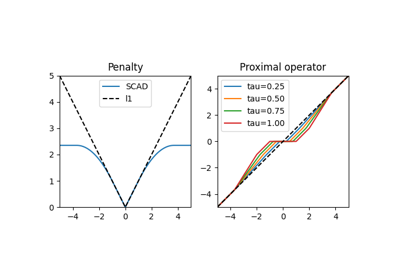Concave penalties

Concave penaltiesNorms

Norms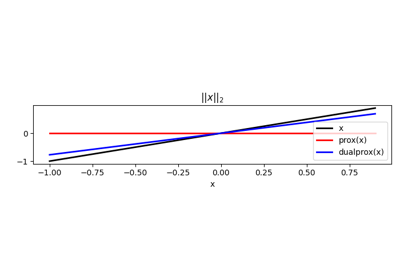Norms

Norms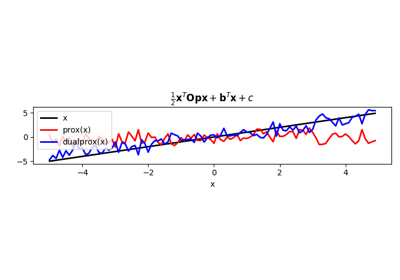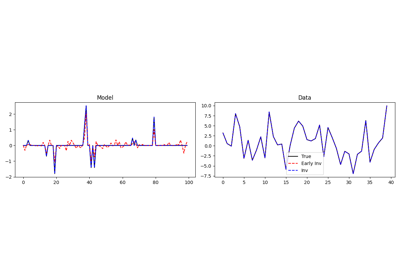Basis Pursuit

Basis Pursuit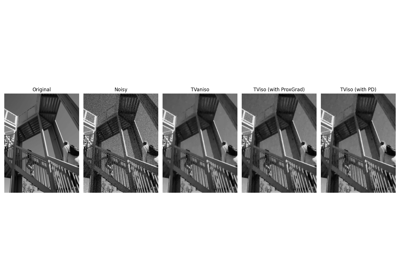Denoising

DenoisingHankel matrix estimation

Hankel matrix estimation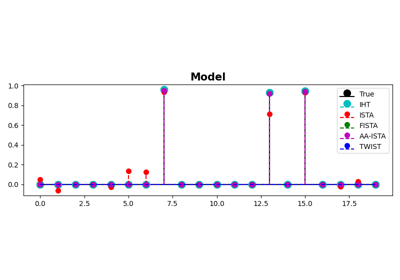ISTA, FISTA, and TWIST for Compressive sensing

ISTA, FISTA, and TWIST for Compressive sensing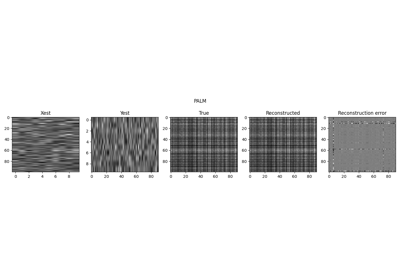Low-Rank completion via Matrix factorization

Low-Rank completion via Matrix factorization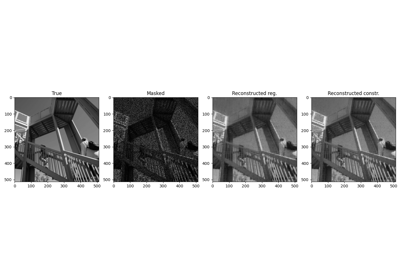Low-Rank completion via SVD

Low-Rank completion via SVD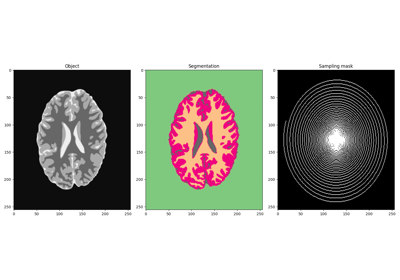MRI Imaging and Segmentation of Brain

MRI Imaging and Segmentation of BrainNon-rigid structure-from-motion (NRSfM)

Non-rigid structure-from-motion (NRSfM)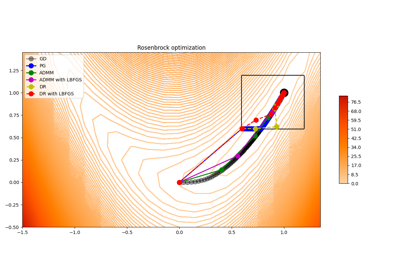Nonlinear inversion with box constraints

Nonlinear inversion with box constraints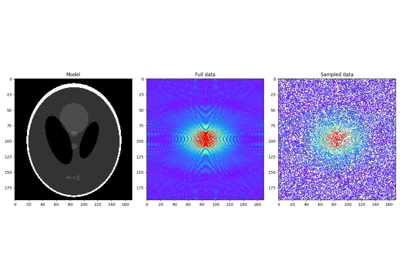Plug and Play Priors

Plug and Play Priors